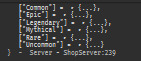# Script only loops through 2 tables?

So this is really weird, my table has 6 elements but only goes through two? This is the code:

``````local function restockRoster()
table.clear(towerRoster)
local hours = tonumber(os.date("%H"))
local days = tonumber(os.date("%j"))
local month = tonumber(os.date("%m"))
local seed = hours * days * month

math.randomseed(seed+10) -- You can use any seed value you prefer
print(towers)
for i, rarityTable in pairs(towers) do
print(rarityTable)
local keys = {}
for key, _ in pairs(rarityTable) do
table.insert(keys, key)
end

if #keys == 1 then
local onlyKey = keys
return rarityTable[onlyKey]
end

local position = math.random(1, #keys)
local key = keys[position]

table.insert(towerRoster,rarityTable[key])

local r1 =  math.random(1, #keys)
local r2 =  math.random(1, #keys)

print(r1,r2)

end
print(towerRoster)
end
``````

The line I print towers, its correctBut when I print the rarityTable it ONLY does Common and Legendary, the “roster” btw is just a blank dictionary in a module. Any help is appreciated, thanks!